This is “The Elements of Group 13”, section 22.1 from the book Principles of General Chemistry (v. 1.0M). For details on it (including licensing), click here.

For more information on the source of this book, or why it is available for free, please see the project's home page. You can browse or download additional books there. To download a .zip file containing this book to use offline, simply click here.

Has this book helped you? Consider passing it on:
Creative Commons supports free culture from music to education. Their licenses helped make this book available to you.
DonorsChoose.org helps people like you help teachers fund their classroom projects, from art supplies to books to calculators.

## 22.1 The Elements of Group 13

### Learning Objective

1. To understand the trends in properties and the reactivity of the group 13 elements.

Group 13 is the first group to span the dividing line between metals and nonmetals, so its chemistry is more diverse than that of groups 1 and 2, which include only metallic elements. Except for the lightest element (boron), the group 13 elements are all relatively electropositive; that is, they tend to lose electrons in chemical reactions rather than gain them. Although group 13 includes aluminum, the most abundant metal on Earth, none of these elements was known until the early 19th century because they are never found in nature in their free state. Elemental boron and aluminum, which were first prepared by reducing B2O3 and AlCl3, respectively, with potassium, could not be prepared until potassium had been isolated and shown to be a potent reductant. Indium (In) and thallium (Tl) were discovered in the 1860s by using spectroscopic techniques, long before methods were available for isolating them. Indium, named for its indigo (deep blue-violet) emission line, was first observed in the spectrum of zinc ores, while thallium (from the Greek thallos, meaning “a young, green shoot of a plant”) was named for its brilliant green emission line. Gallium (Ga; Mendeleev’s eka-aluminum) was discovered in 1875 by the French chemist Paul Émile Lecoq de Boisbaudran during a systematic search for Mendeleev’s “missing” element in group 13.

### Note the Pattern

Group 13 elements are never found in nature in their free state.

## Preparation and General Properties of the Group 13 Elements

As reductants, the group 13 elements are less powerful than the alkali metals and alkaline earth metals. Nevertheless, their compounds with oxygen are thermodynamically stable, and large amounts of energy are needed to isolate even the two most accessible elements—boron and aluminum—from their oxide ores.

Although boron is relatively rare (it is about 10,000 times less abundant than aluminum), concentrated deposits of borax [Na2B4O5(OH)4·8H2O] are found in ancient lake beds (Figure 22.1 "Borax Deposits") and were used in ancient times for making glass and glazing pottery. Boron is produced on a large scale by reacting borax with acid to produce boric acid [B(OH)3], which is then dehydrated to the oxide (B2O3). Reduction of the oxide with magnesium or sodium gives amorphous boron that is only about 95% pure:

Equation 22.1

$Na 2 B 4 O 5 (OH) 4 ⋅ 8 H 2 O(s) → acid B(OH) 3 (s) → Δ B 2 O 3 (s)$

Equation 22.2

Figure 22.1 Borax Deposits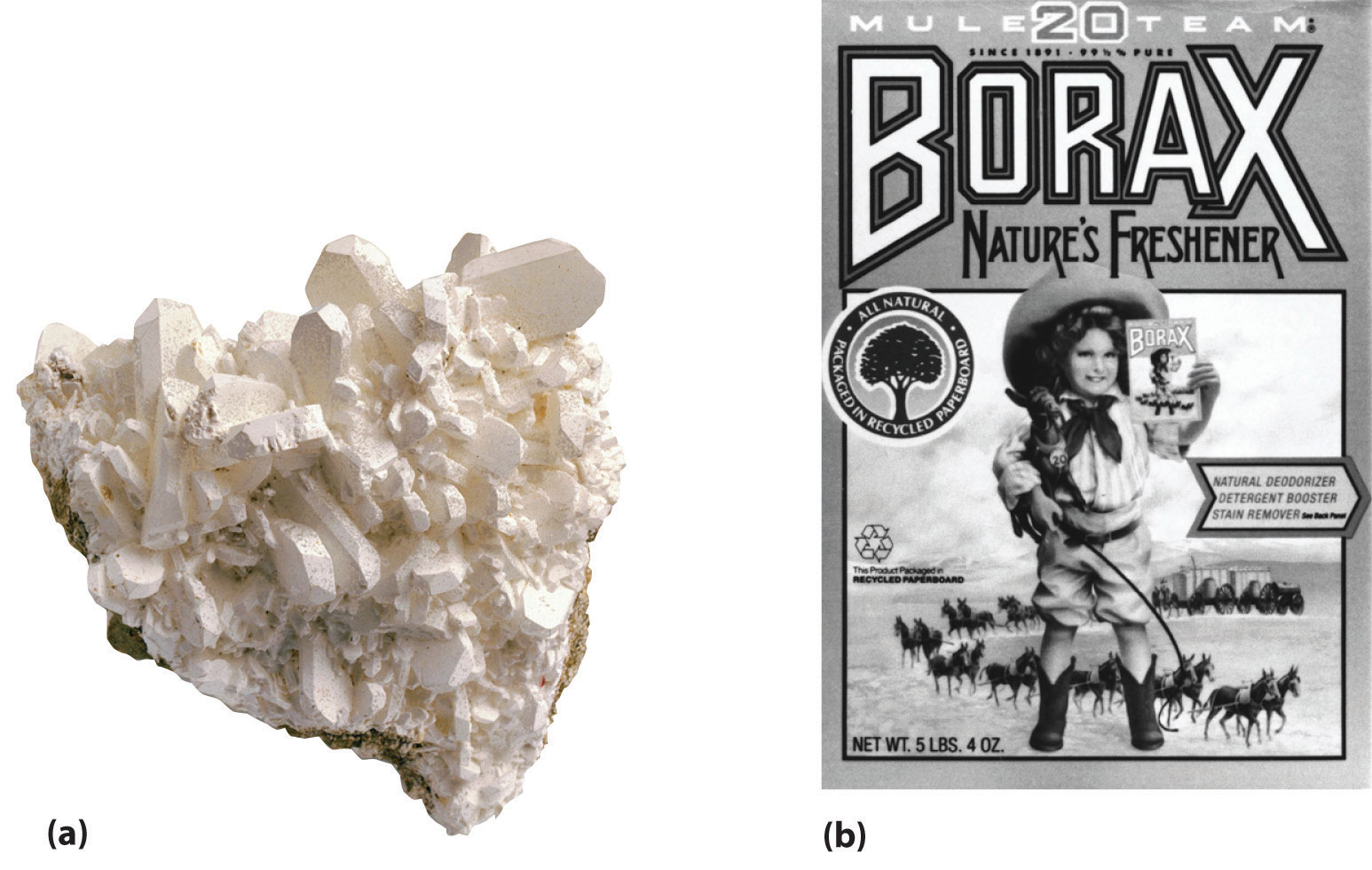(a) Concentrated deposits of crystalline borax [Na2B4O5(OH)4·8H2O] are found in ancient lake beds, such as the Mojave Desert and Death Valley in the western United States. (b) Borax is used in various cleaning products, including 20 Mule Team Borax, a laundry detergent named for the teams of 20 mules that hauled wagons full of borax from desert deposits to railroad terminals in the 1880s.

Pure, crystalline boron, however, is extremely difficult to obtain because of its high melting point (2300°C) and the highly corrosive nature of liquid boron. It is usually prepared by reducing pure BCl3 with hydrogen gas at high temperatures or by the thermal decomposition of boron hydrides such as diborane (B2H6):

Equation 22.3

Equation 22.4

B2H6(g) → 2B(s) + 3H2(g)

The reaction shown in Equation 22.3 is used to prepare boron fibers, which are stiff and light. Hence they are used as structural reinforcing materials in objects as diverse as the US space shuttle and the frames of lightweight bicycles that are used in races such as the Tour de France. Boron is also an important component of many ceramics and heat-resistant borosilicate glasses, such as Pyrex, which is used for ovenware and laboratory glassware.

In contrast to boron, deposits of aluminum ores such as bauxite, a hydrated form of Al2O3, are abundant. With an electrical conductivity about twice that of copper on a weight for weight basis, aluminum is used in more than 90% of the overhead electric power lines in the United States. However, because aluminum–oxygen compounds are stable, obtaining aluminum metal from bauxite is an expensive process. Aluminum is extracted from oxide ores by treatment with a strong base, which produces the soluble hydroxide complex [Al(OH)4]. Neutralization of the resulting solution with gaseous CO2 results in the precipitation of Al(OH)3:

Equation 22.5

2[Al(OH)4](aq) + CO2(g) → 2Al(OH)3(s) + CO32−(aq) + H2O(l)

Thermal dehydration of Al(OH)3 produces Al2O3, and metallic aluminum is obtained by the electrolytic reduction of Al2O3 using the Hall–Heroult process described in Chapter 19 "Electrochemistry". Of the group 13 elements, only aluminum is used on a large scale: for example, each Boeing 777 airplane is about 50% aluminum by mass.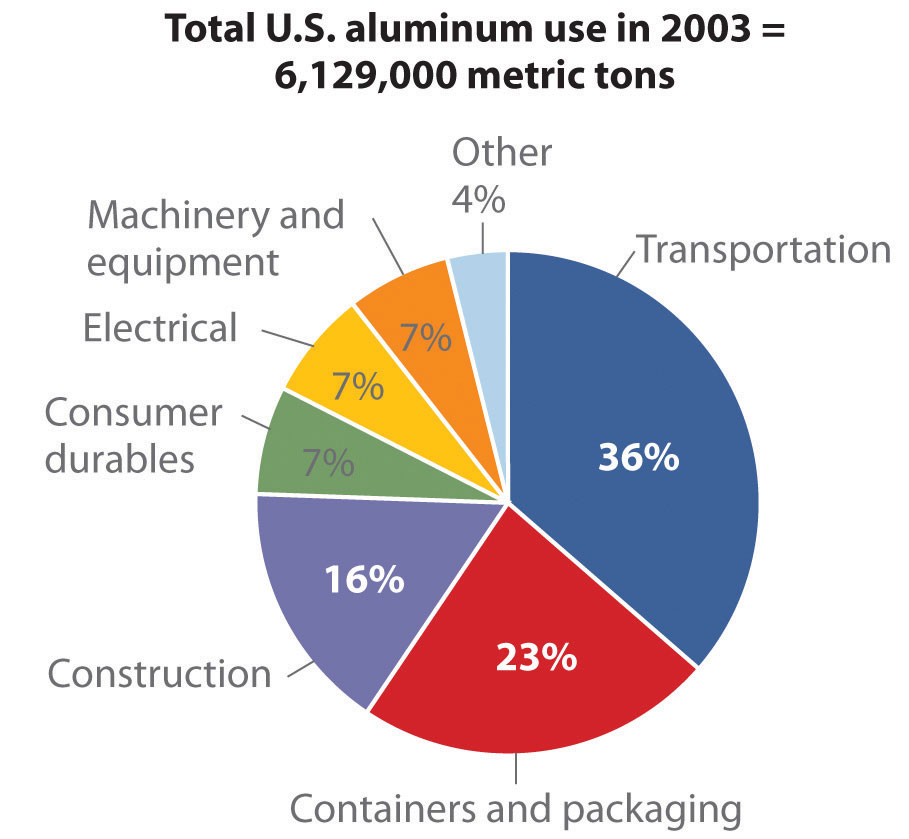The other members of group 13 are rather rare: gallium is approximately 5000 times less abundant than aluminum, and indium and thallium are even scarcer. Consequently, these metals are usually obtained as by-products in the processing of other metals. The extremely low melting point of gallium (29.6°C), however, makes it easy to separate from aluminum. Due to its low melting point and high boiling point, gallium is used as a liquid in thermometers that have a temperature range of almost 2200°C. Indium and thallium, the heavier group 13 elements, are found as trace impurities in sulfide ores of zinc and lead. Indium is used as a crushable seal for high-vacuum cryogenic devices, and its alloys are used as low-melting solders in electronic circuit boards. Thallium, on the other hand, is so toxic that the metal and its compounds have few uses. Both indium and thallium oxides are released in flue dust when sulfide ores are converted to metal oxides and SO2. Until relatively recently, these and other toxic elements were allowed to disperse in the air, creating large “dead zones” downwind of a smelter. The flue dusts are now trapped and serve as a relatively rich source of elements such as In and Tl (as well as Ge, Cd, Te, and As).

Table 22.1 "Selected Properties of the Group 13 Elements" summarizes some important properties of the group 13 elements. Notice the large differences between boron and aluminum in size, ionization energy, electronegativity, and standard reduction potential, which is consistent with the observation that boron behaves chemically like a nonmetal and aluminum like a metal. All group 13 elements have ns2np1 valence electron configurations, and all tend to lose their three valence electrons to form compounds in the +3 oxidation state. The heavier elements in the group can also form compounds in the +1 oxidation state formed by the formal loss of the single np valence electron. Because the group 13 elements generally contain only six valence electrons in their neutral compounds, these compounds are all moderately strong Lewis acids.

Table 22.1 Selected Properties of the Group 13 Elements

Property Boron Aluminum* Gallium Indium Thallium
atomic symbol B Al Ga In Tl
atomic number 5 13 31 49 81
atomic mass (amu) 10.81 26.98 69.72 114.82 204.38
valence electron configuration 2s22p1 3s23p1 4s24p1 5s25p1 6s26p1
melting point/boiling point (°C) 2075/4000 660/2519 29.7/2204 156.6/2072 304/1473
density (g/cm3) at 25°C 2.34 2.70 5.91 7.31 11.8
atomic radius (pm) 87 118 136 156 156
first ionization energy (kJ/mol) 801 578 579 558 589
most common oxidation state +3 +3 +3 +3 +1
ionic radius (pm) −25 54 62 80 162
electron affinity (kJ/mol) −27 −42 −40 −39 −37
electronegativity 2.0 1.6 1.8 1.8 1.8
standard reduction potential (E°, V) −0.87 −1.66 −0.55 −0.34 +0.741 of M3+(aq)
product of reaction with O2 B2O3 Al2O3 Ga2O3 In2O3 Tl2O
type of oxide acidic amphoteric amphoteric amphoteric basic
product of reaction with N2 BN AlN GaN InN none
product of reaction with X2§ BX3 Al2X6 Ga2X6 In2X6 TlX
*This is the name used in the United States; the rest of the world inserts an extra i and calls it aluminium.
The configuration shown does not include filled d and f subshells.
The values cited are for six-coordinate ions in the most common oxidation state, except for Al3+, for which the value for the four-coordinate ion is given. The B3+ ion is not a known species; the radius cited is an estimated four-coordinate value.
§X is Cl, Br, or I. Reaction with F2 gives the trifluorides (MF3) for all group 13 elements.

### Note the Pattern

Neutral compounds of the group 13 elements are electron deficient, so they are generally moderately strong Lewis acids.

In contrast to groups 1 and 2, the group 13 elements show no consistent trends in ionization energies, electron affinities, and reduction potentials, whereas electronegativities actually increase from aluminum to thallium. Some of these anomalies, especially for the series Ga, In, Tl, can be explained by the increase in the effective nuclear charge (Zeff) that results from poor shielding of the nuclear charge by the filled (n − 1)d10 and (n − 2)f14 subshells. Consequently, although the actual nuclear charge increases by 32 as we go from indium to thallium, screening by the filled 5d and 4f subshells is so poor that Zeff increases significantly from indium to thallium. Thus the first ionization energy of thallium is actually greater than that of indium.

### Note the Pattern

Anomalies in periodic trends among Ga, In, and Tl can be explained by the increase in the effective nuclear charge due to poor shielding.

## Reactions and Compounds of Boron

Elemental boron is a semimetal that is remarkably unreactive; in contrast, the other group 13 elements all exhibit metallic properties and reactivity. We therefore consider the reactions and compounds of boron separately from those of other elements in the group.

All group 13 elements have fewer valence electrons than valence orbitals, which generally results in delocalized, metallic bonding. With its high ionization energy, low electron affinity, low electronegativity, and small size, however, boron does not form a metallic lattice with delocalized valence electrons. Instead, boron forms unique and intricate structures that contain multicenter bonds, in which a pair of electrons holds together three or more atoms.

### Note the Pattern

Elemental boron forms multicenter bonds, whereas the other group 13 elements exhibit metallic bonding.

The basic building block of elemental boron is not the individual boron atom, as would be the case in a metal, but rather the B12 icosahedron. (For more information on B12, see Chapter 7 "The Periodic Table and Periodic Trends", Section 7.4 "The Chemical Families".) Because these icosahedra do not pack together very well, the structure of solid boron contains voids, resulting in its low density (Figure 22.2 "Solid Boron Contains B"). Elemental boron can be induced to react with many nonmetallic elements to give binary compounds that have a variety of applications. For example, plates of boron carbide (B4C) can stop a 30-caliber, armor-piercing bullet, yet they weigh 10%–30% less than conventional armor. Other important compounds of boron with nonmetals include boron nitride (BN), which is produced by heating boron with excess nitrogen (Equation 22.6); boron oxide (B2O3), which is formed when boron is heated with excess oxygen (Equation 22.7); and the boron trihalides (BX3), which are formed by heating boron with excess halogen (Equation 22.8).

Equation 22.6

Equation 22.7

Equation 22.8

Figure 22.2 Solid Boron Contains B12 Icosahedra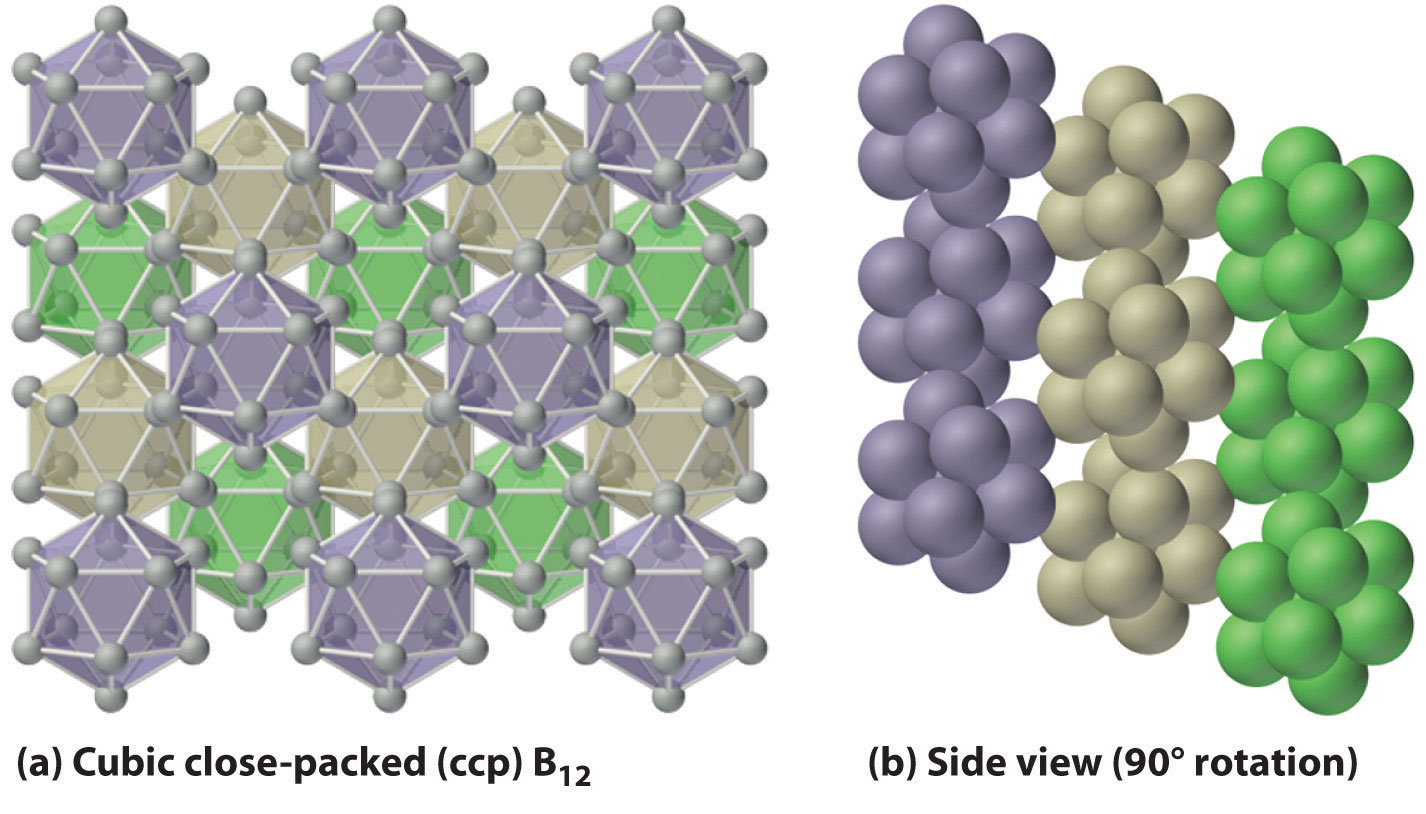Unlike metallic solids, elemental boron consists of a regular array of B12 icosahedra rather than individual boron atoms. Note that each boron atom in the B12 icosahedron is connected to five other boron atoms within the B12 unit. (a) The allotrope of boron with the simplest structure is α-rhombohedral boron, which consists of B12 octahedra in an almost cubic close-packed lattice. (b) A side view of the structure shows that icosahedra do not pack as efficiently as spheres, making the density of solid boron less than expected.

As is typical of elements lying near the dividing line between metals and nonmetals, many compounds of boron are amphoteric, dissolving in either acid or base.

Boron nitride is similar in many ways to elemental carbon. With eight electrons, the B–N unit is isoelectronic with the C–C unit, and B and N have the same average size and electronegativity as C. The most stable form of BN is similar to graphite, containing six-membered B3N3 rings arranged in layers. At high temperature and pressure, hexagonal BN converts to a cubic structure similar to diamond, which is one of the hardest substances known. Boron oxide (B2O3) contains layers of trigonal planar BO3 groups (analogous to BX3) in which the oxygen atoms bridge two boron atoms. It dissolves many metal and nonmetal oxides, including SiO2, to give a wide range of commercially important borosilicate glasses. A small amount of CoO gives the deep blue color characteristic of “cobalt blue” glass.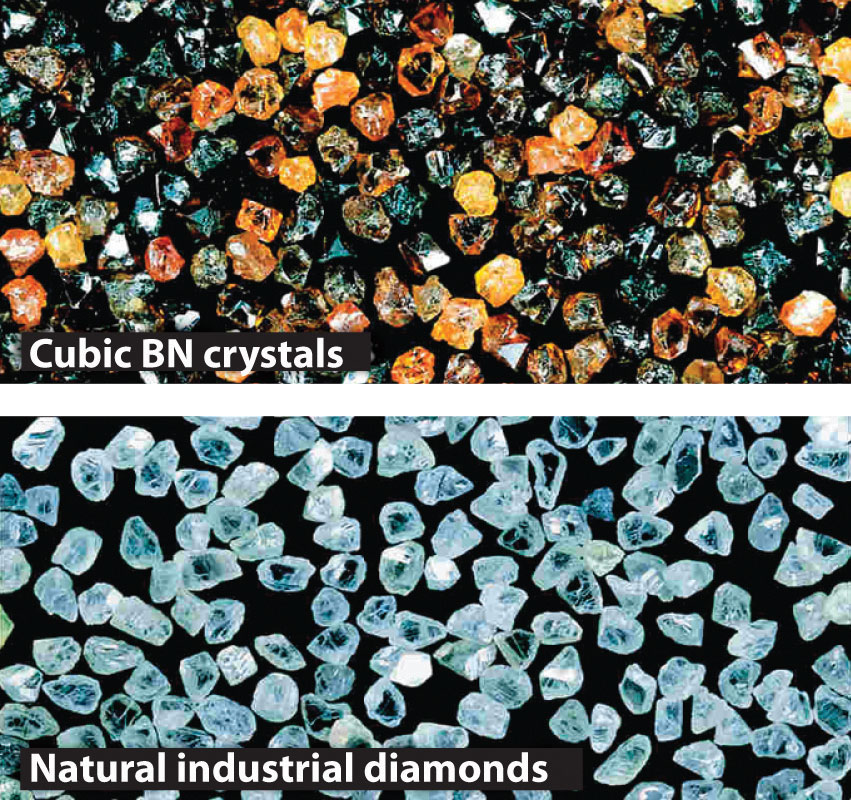At high temperatures, boron also reacts with virtually all metals to give metal borides that contain regular three-dimensional networks, or clusters, of boron atoms. The structures of two metal borides—ScB12 and CaB6—are shown in Figure 22.3 "The Structures of ScB". Because metal-rich borides such as ZrB2 and TiB2 are hard and corrosion resistant even at high temperatures, they are used in applications such as turbine blades and rocket nozzles.

Figure 22.3 The Structures of ScB12 and CaB6, Two Boron-Rich Metal Borides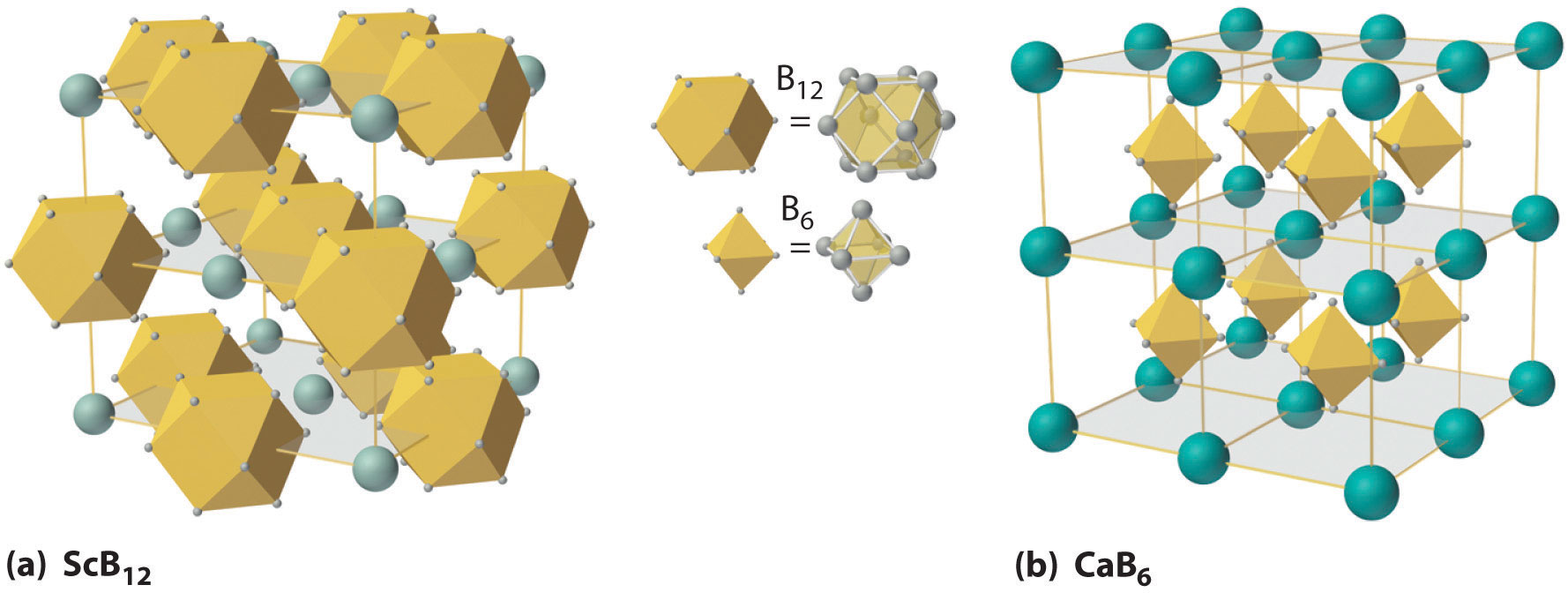(a) The structure of ScB12 consists of B12 clusters and Sc atoms arranged in a faced-centered cubic lattice similar to that of NaCl, with B12 units occupying the anion positions and scandium atoms the cation positions. The B12 units here are not icosahedra but cubooctahedra, with alternating square and triangular faces. (b) The structure of CaB6 consists of octahedral B6 clusters and calcium atoms arranged in a body-centered cubic lattice similar to that of CsCl, with B6 units occupying the anion positions and calcium atoms the cation positions.

Boron hydrides were not discovered until the early 20th century, when the German chemist Alfred Stock undertook a systematic investigation of the binary compounds of boron and hydrogen, although binary hydrides of carbon, nitrogen, oxygen, and fluorine have been known since the 18th century. Between 1912 and 1936, Stock oversaw the preparation of a series of boron–hydrogen compounds with unprecedented structures that could not be explained with simple bonding theories. All these compounds contain multicenter bonds, as discussed in Chapter 21 "Periodic Trends and the " (Figure 21.5 "A Three-Center Bond Uses Two Electrons to Link Three Atoms"). The simplest example is diborane (B2H6), which contains two bridging hydrogen atoms (part (a) in Figure 22.4 "The Structures of Diborane (B"). An extraordinary variety of polyhedral boron–hydrogen clusters is now known; one example is the B12H122− ion, which has a polyhedral structure similar to the icosahedral B12 unit of elemental boron, with a single hydrogen atom bonded to each boron atom.

Figure 22.4 The Structures of Diborane (B2H6) and Aluminum Chloride (Al2Cl6)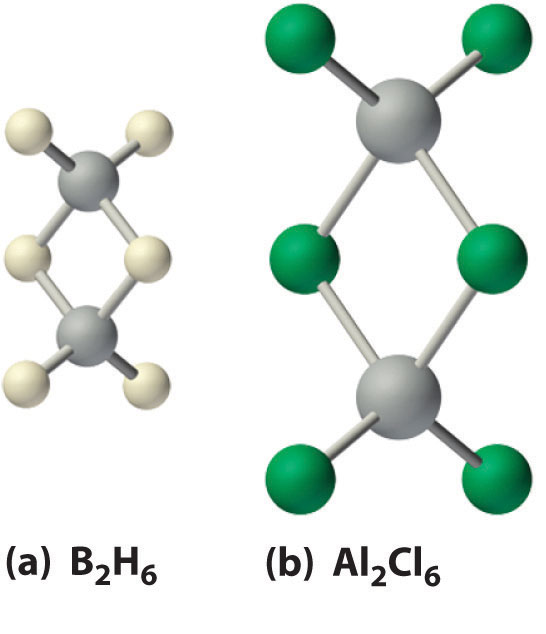(a) The hydrogen-bridged dimer B2H6 contains two three-center, two-electron bonds as described for the B2H7 ion in Figure 21.5 "A Three-Center Bond Uses Two Electrons to Link Three Atoms". (b) In contrast, the bonding in the halogen-bridged dimer Al2Cl6 can be described in terms of electron-pair bonds, in which a chlorine atom bonded to one aluminum atom acts as a Lewis base by donating a lone pair of electrons to another aluminum atom, which acts as a Lewis acid.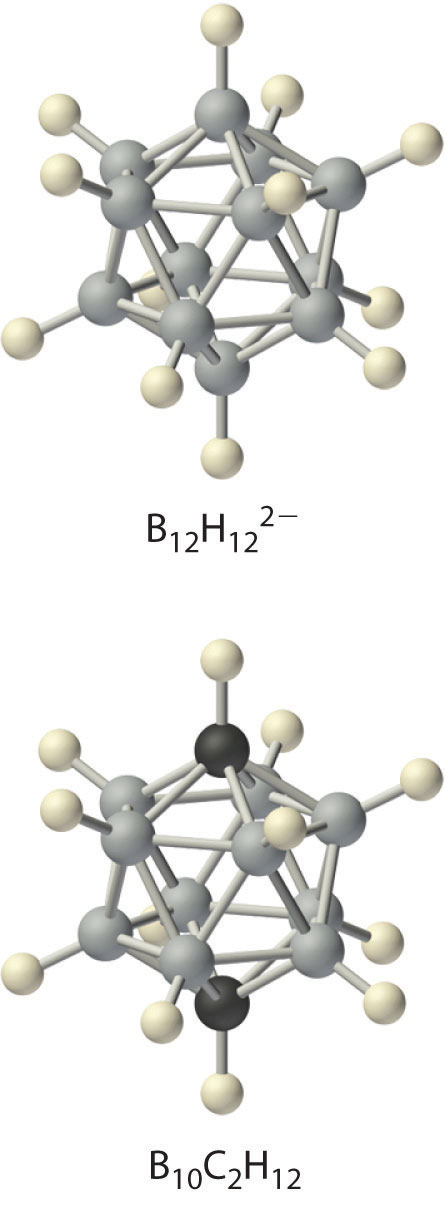A related class of polyhedral clusters, the carboranes, contain both CH and BH units; an example is shown here. Replacing the hydrogen atoms bonded to carbon with organic groups produces substances with novel properties, some of which are currently being investigated for their use as liquid crystals and in cancer chemotherapy.

The enthalpy of combustion of diborane (B2H6) is −2165 kJ/mol, one of the highest values known:

Equation 22.9

Consequently, the US military explored using boron hydrides as rocket fuels in the 1950s and 1960s. This effort was eventually abandoned because boron hydrides are unstable, costly, and toxic, and, most important, B2O3 proved to be highly abrasive to rocket nozzles. Reactions carried out during this investigation, however, showed that boron hydrides exhibit unusual reactivity.

Because boron and hydrogen have almost identical electronegativities, the reactions of boron hydrides are dictated by minor differences in the distribution of electron density in a given compound. In general, two distinct types of reaction are observed: electron-rich species such as the BH4 ion are reductants, whereas electron-deficient species such as B2H6 act as oxidants.

### Example 1

For each reaction, explain why the given products form.

1. B2H6(g) + 3O2(g) → B2O3(s) + 3H2O(l)
2. BCl3(l) + 3H2O(l) → B(OH)3(aq) + 3HCl(aq)

Given: balanced chemical equations

Asked for: why the given products form

Strategy:

Classify the type of reaction. Using periodic trends in atomic properties, thermodynamics, and kinetics, explain why the reaction products form.

Solution:

1. Molecular oxygen is an oxidant. If the other reactant is a potential reductant, we expect that a redox reaction will occur. Although B2H6 contains boron in its highest oxidation state (+3), it also contains hydrogen in the −1 oxidation state (the hydride ion). Because hydride is a strong reductant, a redox reaction will probably occur. We expect that H will be oxidized to H+ and O2 will be reduced to O2−, but what are the actual products? A reasonable guess is B2O3 and H2O, both stable compounds.
2. Neither BCl3 nor water is a powerful oxidant or reductant, so a redox reaction is unlikely; a hydrolysis reaction is more probable. Nonmetal halides are acidic and react with water to form a solution of the hydrohalic acid and a nonmetal oxide or hydroxide. In this case, the most probable boron-containing product is boric acid [B(OH)3].
3. We normally expect a boron trihalide to behave like a Lewis acid. In this case, however, the other reactant is elemental hydrogen, which usually acts as a reductant. The iodine atoms in BI3 are in the lowest accessible oxidation state (−1), and boron is in the +3 oxidation state. Consequently, we can write a redox reaction in which hydrogen is oxidized and boron is reduced. Because compounds of boron in lower oxidation states are rare, we expect that boron will be reduced to elemental boron. The other product of the reaction must therefore be HI.

Exercise

Predict the products of the reactions and write a balanced chemical equation for each reaction.

1. B2H6(g) + H2O(l) $→Δ$
2. BBr3(l) + O2(g) →
3. B2O3(s) + Ca(s) $→Δ$

Answer:

1. B2H6(g) + H2O(l) $→Δ$ 2B(OH)3(s) + 6H2(g)
2. BBr3(l) + O2(g) → no reaction
3. 6B2O3(s) + 18Ca(s) $→Δ$ B12(s) + 18CaO(s)

## Reactions and Compounds of the Heavier Group 13 Elements

All four of the heavier group 13 elements (Al, Ga, In, and Tl) react readily with the halogens to form compounds with a 1:3 stoichiometry:

Equation 22.10

2M(s) + 3X2(s,l,g) → 2MX3(s) or M2X6

The reaction of Tl with iodine is an exception: although the product has the stoichiometry TlI3, it is not thallium(III) iodide, but rather a thallium(I) compound, the Tl+ salt of the triiodide ion (I3). This compound forms because iodine is not a powerful enough oxidant to oxidize thallium to the +3 oxidation state.

Of the halides, only the fluorides exhibit behavior typical of an ionic compound: they have high melting points (>950°C) and low solubility in nonpolar solvents. In contrast, the trichorides, tribromides, and triiodides of aluminum, gallium, and indium, as well as TlCl3 and TlBr3, are more covalent in character and form halogen-bridged dimers (part (b) in Figure 22.4 "The Structures of Diborane (B"). Although the structure of these dimers is similar to that of diborane (B2H6), the bonding can be described in terms of electron-pair bonds rather than the delocalized electron-deficient bonding found in diborane. Bridging halides are poor electron-pair donors, so the group 13 trihalides are potent Lewis acids that react readily with Lewis bases, such as amines, to form a Lewis acid–base adduct:

Equation 22.11

Al2Cl6(soln) + 2(CH3)3N(soln) → 2(CH3)3N:AlCl3(soln)

In water, the halides of the group 13 metals hydrolyze to produce the metal hydroxide [M(OH)3]:

Equation 22.12

MX3(s) + 3H2O(l) → M(OH)3(s) + 3HX(aq)

In a related reaction, Al2(SO4)3 is used to clarify drinking water by the precipitation of hydrated Al(OH)3, which traps particulates. The halides of the heavier metals (In and Tl) are less reactive with water because of their lower charge-to-radius ratio. Instead of forming hydroxides, they dissolve to form the hydrated metal complex ions: [M(H2O)6]3+.

### Note the Pattern

Of the group 13 halides, only the fluorides behave as typical ionic compounds.

### Note the Pattern

Group 13 trihalides are potent Lewis acids that react with Lewis bases to form a Lewis acid–base adduct.

Like boron (Equation 22.7), all the heavier group 13 elements react with excess oxygen at elevated temperatures to give the trivalent oxide (M2O3), although Tl2O3 is unstable:

Equation 22.13

Aluminum oxide (Al2O3), also known as alumina, is a hard, high-melting-point, chemically inert insulator used as a ceramic and as an abrasive in sandpaper and toothpaste. Replacing a small number of Al3+ ions in crystalline alumina with Cr3+ ions forms the gemstone ruby, whereas replacing Al3+ with a mixture of Fe2+, Fe3+, and Ti4+ produces blue sapphires. The gallium oxide compound MgGa2O4 gives the brilliant green light familiar to anyone who has ever operated a xerographic copy machine. All the oxides dissolve in dilute acid, but Al2O3 and Ga2O3 are amphoteric, which is consistent with their location along the diagonal line of the periodic table, also dissolving in concentrated aqueous base to form solutions that contain M(OH)4 ions.

Aluminum, gallium, and indium also react with the other group 16 elements (chalcogens) to form chalcogenides with the stoichiometry M2Y3. However, because Tl(III) is too strong an oxidant to form a stable compound with electron-rich anions such as S2−, Se2−, and Te2−, thallium forms only the thallium(I) chalcogenides with the stoichiometry Tl2Y. Only aluminum, like boron, reacts directly with N2 (at very high temperatures) to give AlN, which is used in transistors and microwave devices as a nontoxic heat sink because of its thermal stability; GaN and InN can be prepared using other methods. All the metals, again except Tl, also react with the heavier group 15 elements (pnicogens) to form the so-called III–V compounds, such as GaAs. These are semiconductors, whose electronic properties, such as their band gaps, differ from those that can be achieved using either pure or doped group 14 elements. (For more information on band gaps, see Chapter 12 "Solids", Section 12.6 "Bonding in Metals and Semiconductors".) For example, nitrogen- and phosphorus-doped gallium arsenide (GaAs1−xyPxNy) is used in the displays of calculators and digital watches.

### Note the Pattern

All group 13 oxides dissolve in dilute acid, but Al2O3 and Ga2O3 are amphoteric.

Unlike boron, the heavier group 13 elements do not react directly with hydrogen. Only the aluminum and gallium hydrides are known, but they must be prepared indirectly; AlH3 is an insoluble, polymeric solid that is rapidly decomposed by water, whereas GaH3 is unstable at room temperature.

## Complexes of Group 13 Elements

Boron has a relatively limited tendency to form complexes, but aluminum, gallium, indium, and, to some extent, thallium form many complexes. Some of the simplest are the hydrated metal ions [M(H2O)63+], which are relatively strong Brønsted–Lowry acids that can lose a proton to form the M(H2O)5(OH)2+ ion:

Equation 22.14

[M(H2O)6]3+(aq) → M(H2O)5(OH)2+(aq) + H+(aq)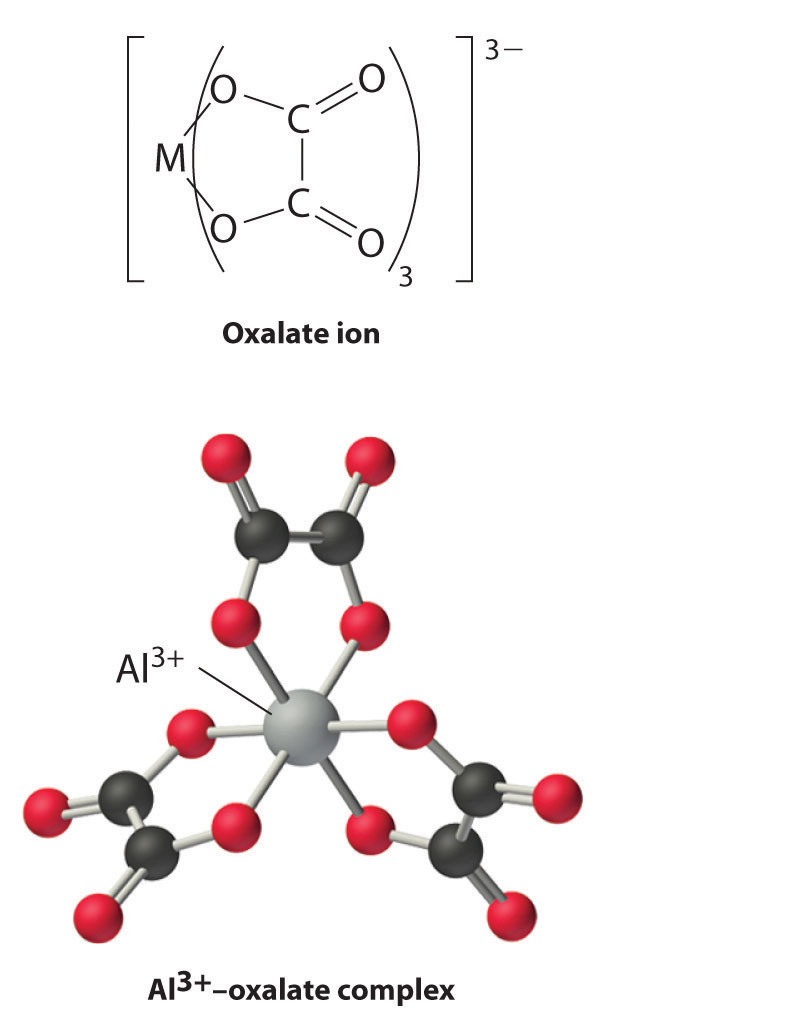Group 13 metal ions also form stable complexes with species that contain two or more negatively charged groups, such as the oxalate ion. The stability of such complexes increases as the number of coordinating groups provided by the ligand increases.

### Example 2

For each reaction, explain why the given products form.

1. 2Al(s) + Fe2O3(s) $→Δ$ 2Fe(l) + Al2O3(s)
2. 2Ga(s) + 6H2O(l) + 2OH(aq) $→Δ$ 3H2(g) + 2Ga(OH)4(aq)
3. In2Cl6(s) $→H2O(l)$ 2In3+(aq) + 6Cl(aq)

Given: balanced chemical equations

Asked for: why the given products form

Strategy:

Classify the type of reaction. Using periodic trends in atomic properties, thermodynamics, and kinetics, explain why the reaction products form.

Solution:

1. Aluminum is an active metal and a powerful reductant, and Fe2O3 contains Fe(III), a potential oxidant. Hence a redox reaction is probable, producing metallic Fe and Al2O3. Because Al is a main group element that lies above Fe, which is a transition element, it should be a more active metal than Fe. Thus the reaction should proceed to the right. In fact, this is the thermite reaction, which is so vigorous that it produces molten Fe and can be used for welding. (For more information on the thermite reaction, see Chapter 5 "Energy Changes in Chemical Reactions", Section 5.2 "Enthalpy".)
2. Gallium lies immediately below aluminum in the periodic table and is amphoteric, so it will dissolve in either acid or base to produce hydrogen gas. Because gallium is similar to aluminum in many of its properties, we predict that gallium will dissolve in the strong base.
3. The metallic character of the group 13 elements increases with increasing atomic number. Indium trichloride should therefore behave like a typical metal halide, dissolving in water to form the hydrated cation.

Exercise

Predict the products of the reactions and write a balanced chemical equation for each reaction.

1. LiH(s) + Al2Cl6(soln)→
2. Al2O3(s) + OH(aq)→
3. Al(s) + N2(g) $→Δ$
4. Ga2Cl6(soln) + Cl(soln)→

Answer:

1. 8LiH(s) + Al2Cl6(soln)→2LiAlH4(soln) + 6LiCl(s)
2. Al2O3(s) + 2OH(aq) + 3H2O(l) → 2Al(OH)4(aq)
3. 2Al(s) + N2(g) $→Δ$ 2AlN(s)
4. Ga2Cl6(soln) + 2Cl(soln) → 2GaCl4(soln)

### Summary

Isolation of the group 13 elements requires a large amount of energy because compounds of the group 13 elements with oxygen are thermodynamically stable. Boron behaves chemically like a nonmetal, whereas its heavier congeners exhibit metallic behavior. Many of the inconsistencies observed in the properties of the group 13 elements can be explained by the increase in Zeff that arises from poor shielding of the nuclear charge by the filled (n − 1)d10 and (n − 2)f14 subshells. Instead of forming a metallic lattice with delocalized valence electrons, boron forms unique aggregates that contain multicenter bonds, including metal borides, in which boron is bonded to other boron atoms to form three-dimensional networks or clusters with regular geometric structures. All neutral compounds of the group 13 elements are electron deficient and behave like Lewis acids. The trivalent halides of the heavier elements form halogen-bridged dimers that contain electron-pair bonds, rather than the delocalized electron-deficient bonds characteristic of diborane. Their oxides dissolve in dilute acid, although the oxides of aluminum and gallium are amphoteric. None of the group 13 elements reacts directly with hydrogen, and the stability of the hydrides prepared by other routes decreases as we go down the group. In contrast to boron, the heavier group 13 elements form a large number of complexes in the +3 oxidation state.

### Key Takeaways

• Compounds of the group 13 elements with oxygen are thermodynamically stable.
• Many of the anomalous properties of the group 13 elements can be explained by the increase in Zeff moving down the group.

### Conceptual Problems

1. None of the group 13 elements was isolated until the early 19th century, even though one of these elements is the most abundant metal on Earth. Explain why the discovery of these elements came so late and describe how they were finally isolated.

2. Boron and aluminum exhibit very different chemistry. Which element forms complexes with the most ionic character? Which element is a metal? a semimetal? What single property best explains the difference in their reactivity?

3. The usual oxidation state of boron and aluminum is +3, whereas the heavier elements in group 13 have an increasing tendency to form compounds in the +1 oxidation state. Given that all group 13 elements have an ns2np1 electron configuration, how do you explain this difference between the lighter and heavier group 13 elements?

4. Do you expect the group 13 elements to be highly reactive in air? Why or why not?

5. Which of the group 13 elements has the least metallic character? Explain why.

6. Boron forms multicenter bonds rather than metallic lattices with delocalized valence electrons. Why does it prefer this type of bonding? Does this explain why boron behaves like a semiconductor rather than a metal? Explain your answer.

7. Because the B–N unit is isoelectronic with the C–C unit, compounds that contain these units tend to have similar chemistry, although they exhibit some important differences. Describe the differences in physical properties, conductivity, and reactivity of these two types of compounds.

8. Boron has a strong tendency to form clusters. Does aluminum have this same tendency? Why or why not?

9. Explain why a B–O bond is much stronger than a B–C bond.

10. The electron affinities of boron and aluminum are −27 and −42 kJ/mol, respectively. Contrary to the usual periodic trends, the electron affinities of the remaining elements in group 13 are between those of B and Al. How do you explain this apparent anomaly?

11. The reduction potentials of B and Al in the +3 oxidation state are −0.87 V and −1.66 V, respectively. Do you expect the reduction potentials of the remaining elements of group 13 to be greater than or less than these values? How do you explain the differences between the expected values and those given in Table 22.1 "Selected Properties of the Group 13 Elements"?

12. The compound Al2Br6 is a halide-bridged dimer in the vapor phase, similar to diborane (B2H6). Draw the structure of Al2Br6 and then compare the bonding in this compound with that found in diborane. Explain the differences.

13. The compound AlH3 is an insoluble, polymeric solid that reacts with strong Lewis bases, such as Me3N, to form adducts with 10 valence electrons around aluminum. What hybrid orbital set is formed to allow this to occur?

### Answers

1. The high stability of compounds of the group 13 elements with oxygen required powerful reductants such as metallic potassium to be isolated. Al and B were initially prepared by reducing molten AlCl3 and B2O3, respectively, with potassium.

2. Due to its low electronegativity and small size, boron is an unreactive semimetal rather than a metal.

3. The B–N bond is significantly more polar than the C–C bond, which makes B–N compounds more reactive and generally less stable than the corresponding carbon compounds. Increased polarity results in less delocalization and makes the planar form of BN less conductive than graphite.

4. Partial pi bonding between O and B increases the B–O bond strength.

5. Periodic trends predict that the cations of the heavier elements should be easier to reduce, so the elements should have less negative reduction potentials. In fact, the reverse is observed because the heavier elements have anomalously high Zeff values due to poor shielding by filled (n − 1)d and (n − 2)f subshells.

6. dsp 3

### Structure and Reactivity

1. Is B(OH)3 a strong or a weak acid? Using bonding arguments, explain why.

2. Using bonding arguments, explain why organoaluminum compounds are expected to be potent Lewis acids that react rapidly with weak Lewis bases.

3. Imagine that you are studying chemistry prior to the discovery of gallium, element 31. Considering its position in the periodic table, predict the following properties of gallium:

1. chemical formulas of its most common oxide, most common chloride, and most common hydride
2. solubility of its oxide in water and the acidity or basicity of the resulting solution
3. the principal ion formed in aqueous solution
4. The halides of Al, Ga, In, and Tl dissolve in water to form acidic solutions containing the hydrated metal ions, but only the halides of aluminum and gallium dissolve in aqueous base to form soluble metal-hydroxide complexes. Show the formulas of the soluble metal–hydroxide complexes and of the hydrated metal ions. Explain the difference in their reactivities.

5. Complete and balance each chemical equation.

1. BCl3(g) + H2(g) $→Δ$
2. 6C2H4(g) + B2H6(g) →
3. B2H6(g) + 3Cl2(g) →
4. B2H6(g) + 2(C2H5)2S(g) →
6. Complete and balance each chemical equation.

1. BBr3(g) + H2(g) $→Δ$
2. BF3(g) + F(g) →
3. LiH(s) + B2H6(g) →
4. B(OH)3(s) + NaOH(aq) →
7. Write a balanced chemical equation for each reaction.

1. the dissolution of Al2O3 in dilute acid
2. the dissolution of Ga2O3 in concentrated aqueous base
3. the dissolution of Tl2O in concentrated aqueous acid
4. Ga(l) + S8(s)
5. Tl(s) + H2S(g)
8. Write a balanced chemical equation for the reaction that occurs between Al and each species.

1. Cl2
2. O2
3. S
4. N2
5. H2O
6. H+(aq)
9. Write a balanced chemical equation that shows how you would prepare each compound from its respective elements or other common compounds.

1. In2I6
2. B(OH)3
3. Ga2O3
4. [Tl(H2O)6]3+
5. Al(OH)4
6. In4C3
10. Write a balanced chemical equation that shows how you would prepare each compound from its respective elements or other common compounds.

1. BCl3
2. InCl3
3. Tl2S
4. Al(OH)3
5. In2O3
6. AlN
11. Diborane is a spontaneously flammable, toxic gas that is prepared by reacting NaBH4 with BF3. Write a balanced chemical equation for this reaction.

12. Draw the Lewis electron structure of each reactant and product in each chemical equation. Then describe the type of bonding found in each reactant and product.

1. 2B(s) + 3X2(g) $→Δ$ 2BX3(g)
2. 4B(s) + 3O2(g) $→Δ$ 2B2O3(s)
3. 2B(s) + N2(g) $→Δ$ 2BN(s)
13. Draw the Lewis electron structure of each reactant and product in each chemical equation. Then describe the type of bonding found in each reactant and product.

1. B2H6(g) + 3O2(g) → B2O3(s) + 3H2O(l)
2. B2H6(g) + 6CH3CH=CH2(g) → 2[B(CH2CH2CH3)3](l)

### Answers

1. 12BCl3(g) + 18H2(g) $→Δ$B12(s) + 36HCl(g)
2. 6C2H4(g) + B2H6(g) → 2B(C2H5)3(l)
3. 6B2H6(g) + 18Cl2(g) → B12(s) + 36HCl(g)
4. B2H6(g) + 2(C2H5)2S(g) → 2H3B·S(C2H5)2(l)
1. Al2O3(s) + 6H3O+(aq) + 3H2O(l) → 2Al(H2O)63+(aq)
2. Ga2O3(s) + 2OH(aq) + 3H2O(l) → 2Ga(OH)4(aq)
3. Tl2O(s) + 2H+(aq) + 9H2O(l) → 2Tl(H2O)6+(aq)
4. 16Ga(l) + 3S8(s) → 8Ga2S3(s)
5. 2Tl(s) + H2S(g) → Tl2S(s) + H2(g)
1. 2In(s) + 3I2(s) $→Δ$ In2I6(s)
2. B12(s) + 18Cl2(g) → 12BCl3(l)

BCl3(l) + 3H2O(l) → B(OH)3(aq) + 3HCl(aq)

3. 4Ga(l) + 3O2(g) $→Δ$ 2Ga2O3(s)
4. 2Tl(s) + 3Cl2(g) → 2TlCl3(s); TlCl3(s) + 6H2O(l) → Tl(H2O6)3+(aq) + 3Cl(aq)
5. 2Na(s) + 2H2O(l) → 2NaOH(aq) + H2(g); 2Al(s) + 2NaOH(aq) + 6H2O(l) → 2Al(OH)4 + 3H2(g) + 2Na+(aq)
6. 4In(s) + 3C(s) $→Δ$ In4C3(s)